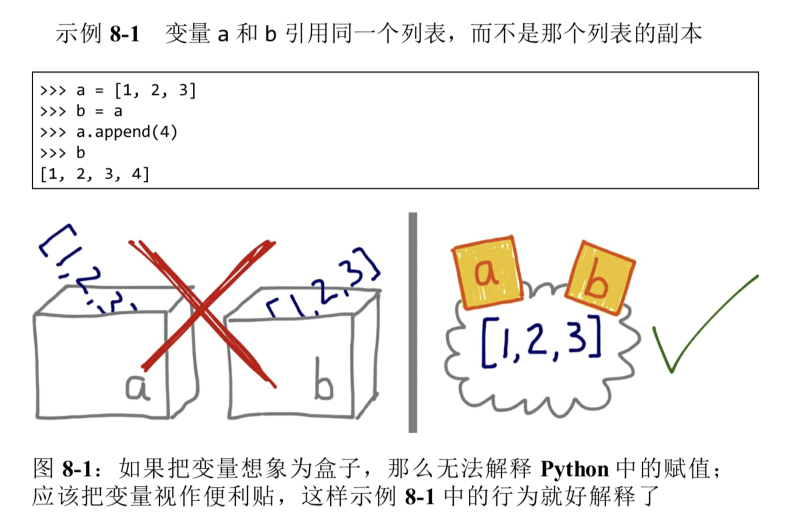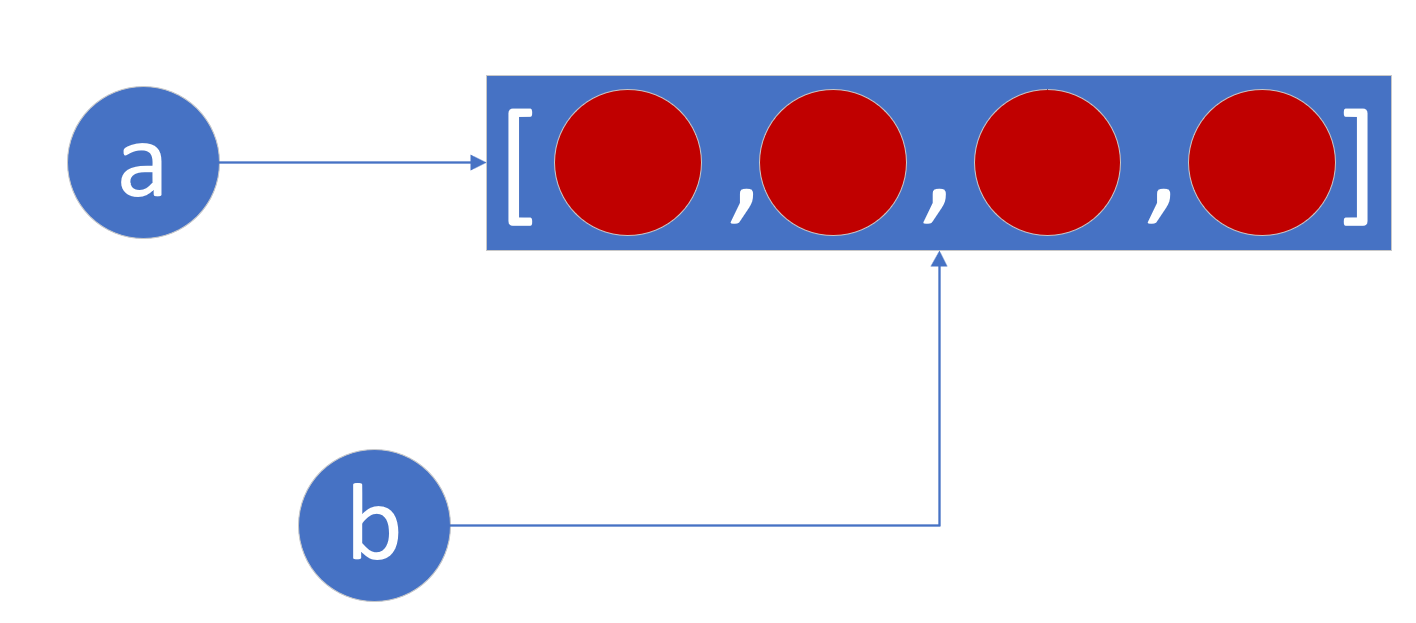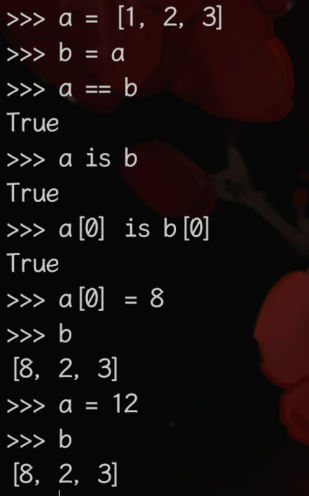# Python里的引用与拷贝规律

2022-04-05 16:19 211 查看

python的可变不可变与各种浅拷贝深拷贝规则，一并梳理。

### Python一切皆引用

`int a = 1`

`int b = a``a = [1, 2, 3]`

`[1,2,3]`
，再让a来代表它；
`b = a`

`del a`
），也仅仅是撕下来一张标签而已，b仍然可以访问这个列表。其他类型也是如此

### 情况一：直接引用`b = a`
，正如上文所说，不会发生拷贝，只是让b也来代表a代表的区域。此时b就是a，b也就是a。`a = a + b`

`a = (1, 2, 3); b = a; a += (4, 5)`
，此时执行
`a = a + (4, 5)`
，已经指向新的值了，所以b不会改变。

### 情况二：复制

```b = a[:]
b = list(_ for _ in a)
b = copy(a)
b = a.copy()```

`a is b`

### 情况三：深复制

```from copy import deepcopy
a = [1, [1, 2, 3], "hello"]
b = deepcopy(a)```